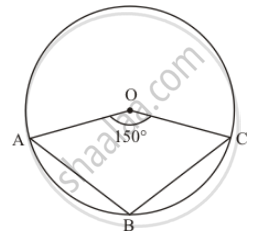Advertisement Remove all ads

# In the Given Figure, It is Given that O is the Centre of the Circle and ∠Aoc = 150°. Find ∠Abc. - Mathematics

Short Note

In the given figure, it is given that O is the centre of the circle and ∠AOC = 150°. Find ∠ABC.Advertisement Remove all ads

#### Solution

It is given that O is the centre of circle and AB and C are points on circumference.

angle AOC = 150°  (Given)We have to find ABC

The angle subtended by an arc of a circle at the centre is double the angle subtended by it at any point on the remaining part of the circle.

$\angle ABC = \frac{1}{2}\left( \text{ reflex } \angle AOC \right)$
$= \frac{1}{2}\left( 360° - 150° \right)$
$= \frac{1}{2} \times 210°$
$= 105°$

Hence,angle ABC = 105°

Is there an error in this question or solution?
Advertisement Remove all ads

#### APPEARS IN

RD Sharma Mathematics for Class 9
Chapter 15 Circles
Exercise 15.4 | Q 9 | Page 74
Advertisement Remove all ads

#### Video TutorialsVIEW ALL 

Advertisement Remove all ads
Share
Notifications

View all notifications

Forgot password?
Course